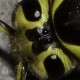Sreejith U -3 years ago 136
C++ Question

# How to create a queue of function pointers in C++

I am trying to create a co-operative scheduler using C++, for which i need a queue that will contain function pointers.

Does the C++ queue STL library help in this context?Jerry Coffin
Answer Source

This is obviously the exact sort of problem `std::queue` was intended to help solve.

I would change a few things though. One would be to store an `std::function` instead of a raw pointer to a function:

``````struct task {
int id;
std::function<void()> f;
};
``````

This allows you to pass essentially anything that can be invoked like a function, not just a pointer to an actual function. For one obvious example, you can use a lambda expression:

``````    task t { 1, [] {cout << "having fun!\n"; } };
q.push(t);
auto tsk = q.front();
tsk.f();
``````

Since nearly the only thing you can do with a task is to invoke it, I'd also consider providing `task` with an overloaded `operator()` to do the invocation: `void operator()() { f(); }`, so if you just want to invoke a task, you could do something like this:

``````auto f = q.front();
f();
``````

Your test program expanded to include these might look more like this:

``````#include <iostream>
#include <queue>
#include <functional>

using namespace std;

struct task {
int id;
std::function<void()> f;

void operator()() { f(); }
};

queue<struct task> q;

void fun(void) {
cout << "Having fun!" << endl;
}

int main() {

cout << "Creating a task object" << endl;

task t;
t.id = 1;
t.f = &fun;

cout << "Calling function directly from object" << endl;
t.f();

cout << "adding the task into the queue" << endl;
q.push(t);

cout << "calling the function from the queue" << endl;
task tsk = q.front();
tsk.f();
q.pop();

q.push({ 1, [] {std::cout << "Even more fun\n"; } });

auto t2 = q.front();
t2.f(); // invoke conventionally

t2();   // invoke via operator()

q.pop();
}
``````
Recommended from our users: Dynamic Network Monitoring from WhatsUp Gold from IPSwitch. Free Download
Latest added